# Algebra - math word problems

#### Number of problems found: 2866

• Divisors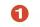Find all divisors of number 493. How many are them?
• Motion2Cyclist started out of town at 19 km/h. After 0.7 hours car started behind him in the same direction and caught up with him for 23 minutes. How fast and how long went car from the city to caught cyclist?
• Cone A2VSurface of cone in the plane is a circular arc with central angle of 126° and area 415 cm2. Calculate the volume of a cone.
• RightDetermine angles of the right triangle with the hypotenuse c and legs a, b, if: ?
• Euclid3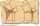Calculate height and sides of the right triangle, if one leg is a = 81 cm and section of hypotenuse adjacent to the second leg cb = 39 cm.
• Right isoscelesCalculate area of the isosceles right triangle which perimeter is 41 cm.
• Euclid1Right triangle has hypotenuse c = 27 cm. How large sections cuts height hc=3 cm on the hypotenuse c?
• Square diagonalCalculate the length of diagonal of the square with side a = 23 cm.
• Lines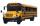Five bus lines runs at intervals 3, 6, 9, 12, 15 minutes. In the morning, suddenly start at 4:00. After how many minutes the bus lines meet again?
• Purchase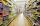Three buyers pay € 468. The first paid 3-times more than second, third half over second. How many euros paid each of them?
• CirclesThe areas of the two circles are in the ratio 2:20. The larger circle has diameter 20. Calculate the radius of the smaller circle.
• NumbersDetermine the number of all positive integers less than 4183444 if each is divisible by 29, 7, 17. What is its sum?
• LinesIn how many points will intersect 14 different lines, where no two are parallel?
• Sequence 3Write the first 5 members of an arithmetic sequence: a4=-35, a11=-105.
• Balance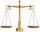The rod are 1.9 m long hanging weights 4 kg and 1 kg on ends. Where are centre of rod (distance from weight 4 kg) to be in balance?
• Sequence 2Write the first 5 members of an arithmetic sequence a11=-14, d=-1
• Gear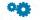Two gears, fit into each other, has transfer 2:3. Centres of gears are spaced 82 cm. What are the radii of the gears?
• SequenceWrite the first 7 members of an arithmetic sequence: a1=-3, d=6.
• Resistors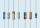Two resistors connected in series give the resulting resistance 65Ω and 10.4Ω in parallel. Determine the resistance of these resistors.
• Cubes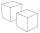Surfaces of cubes, one of which has an edge of 48 cm shorter than the other, differ by 36288 dm2. Determine the length of the edges of this cubes.

Do you have an interesting mathematical word problem that you can't solve it? Submit a math problem, and we can try to solve it.

We will send a solution to your e-mail address. Solved examples are also published here. Please enter the e-mail correctly and check whether you don't have a full mailbox.

Please do not submit problems from current active competitions such as Mathematical Olympiad, correspondence seminars etc...